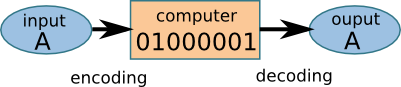### Vocabulary

bit
Binary digit – can be 0 or 1

### Computer Memory

Computers encode and store information as a sequence of bits. This diagram shows how the letter A is encoded.### Reviewing Decimal Numbers

Decimal numbers are base 10. A decimal digit can be 0, 1, 2, 3, 4, 5, 6, 7, 8, or 9.

756 =
7 × 100
+ 5 ×   10
+ 6 ×     1
700
+ 50
+   6

Place Value of Decimal Numbers uses powers of 10.

 106 105 104 103 102 101 100 1,000,000 100,000 10,000 1,000 100 10 1

### Binary Numbers

Binary numbers are base 2. A binary digit can be 0 or 1

101 =
1 × 4
+ 0 × 2
+ 1 × 1
4
+0
+1
5

Place Value of Binary Numbers uses powers of 2.

 26 25 24 23 22 21 20 64 32 16 8 4 2 1

Use the place value table to convert from binary to decimal.

Example 1: Convert the binary number 1011 to decimal.

 64 32 16 8 4 2 1 1 0 1 1 1 × 8 = 8 0 × 4 = 0 1 × 2 = 2 1 × 1 = 1 8  + 0  + 2  + 1 =    11

Example 2: Convert the binary number 110110 to decimal.

 64 32 16 8 4 2 1 1 1 0 1 1 0 1 × 32 = 32 1 × 16 = 16 0 × 8 = 0 1 × 4 = 4 1 × 2 = 2 0 × 1 = 0 32  + 16  + 0  + 4  + 2  + 0 =    54

Adding binary numbers is just like adding decimal numbers, except that you use the binary addition table.

 0 + 0 = 0 0 + 1 = 1 1 + 0 = 1 1 + 1 = 10 1 + 1 + 1 = 11

Example 1: Add 101 + 10

 1 0 1 + 1 0 1 1 1

Example 2: Add 1101 + 11

When you add 1 + 1, you need to carry 1 to the next place.

 1 1 ← carry digits 1 0 1 1 + 1 1 1 1 1 0
Computer Programming Unit 1.2 - How Binary Numbers are Used in Computers